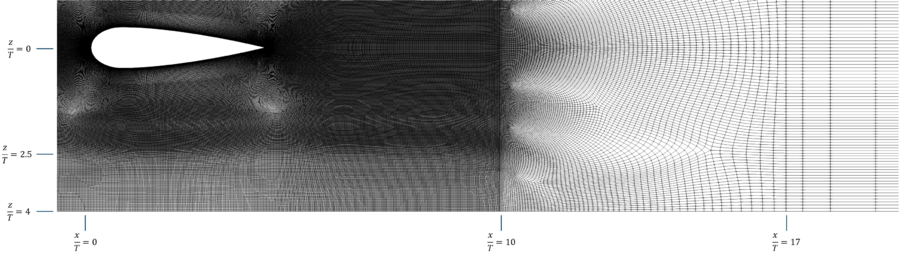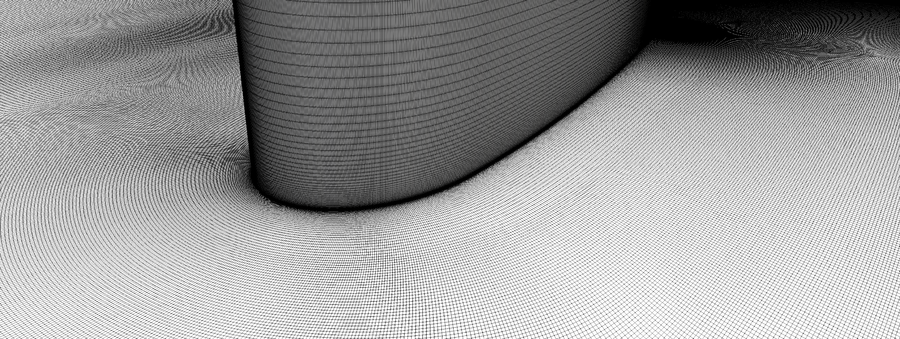# Computational Details

This section provides details of the computational approach used for the simulation of the present flow problem. First, the main numerical features of the code MIGALE are given. Then, information about the computational grid is provided. Finally, the statistical quantities and their computation are explained.

## Computational approach

The CFD code MIGALE can solve both compressible and incompressible flow problems and implements different flow models ranging from the Euler to the RANS equations. The solver uses the Discontinuous Galerkin (DG) method for the spatial discretization of the governing equations, here the compressible Navier-Stokes equations. The discontinuous nature of the numerical solution requires introducing a special treatment of the inviscid interface flux and of the viscous flux. For the former it is common practice to use suitably defined numerical flux functions, which ensure conservation and account for wave propagation. To this purpose, in the compressible case, the exact Riemann solver of Gottlieb et al. (1988) is mainly used. For the latter, the 2nd-scheme of Bassi and Rebay (BR2) is employed, see Bassi et al. (1997). The devised method uses hierarchical and orthonormal polynomial basis functions defined in the physical (mesh) space and relies for the time integration on, primarily, high-order Rosenbrock linearly-implicit schemes, see Bassi et al. (2015). The Jacobian matrix of the spatial discretization is computed analytically and linear systems are solved using preconditioned GMRES methods from the PETSc library (Portable Extensible Toolkit for Scientific Computations). For production runs time integration is coupled with time-step adaptation strategies able to improve the robustness and the efficiency in terms of time-to-solution of simulations, see Noventa et al. (2020).

For the present computation the fourth-order DG spatial discretization is combined with the fifth-order eight stages Rosenbrock-type scheme of Di Marzo (1993).

## Spatial and temporal resolution, grids

The computational grid is made of $53\,937\,564$elements with quadratic edges, resulting in more than 1 billion Degrees of Freedom (DoF) per equation. Fig. 4 illustrates the surface mesh at the bottom boundary from the inlet (left) to the outlet (right). Clearly visible is the mesh coarsening used downstream of the wing to reduce the solution gradients, thus mitigating spurious perturbations possibly originating from the outlet boundary. The coarsening was applied in two steps: (i) both along the span and stream direction for $10(solid wall boundary condition) and (ii) only along the streamwise direction for $x/T>17$(symmetry boundary condition). The surface mesh detail shown in Fig. 5 also reveals a mesh density reduction in the span direction towards the lateral boundaries for $\pm 2.5. This choice was intended to alleviate the large computational cost of the simulation by limiting the fine mesh region to the proximity of the wing. The surface mesh at the floor was stretched in the direction normal to the bottom boundary with a growth factor equal to $1.15$, see Fig. 6.Figure 4: Surface mesh at the bottom boundary (top view)Figure 5: Detail of Surface mesh at the bottom boundary (top view)Figure 6: Detail of the computational grid close to the junction

For the temporal integration, a fifth order linearly implicit Rosenbrock scheme is applied with an adaptive time stepping technique. The resulting average time step is roughly $\Delta t\approx 1/398\,CTU$, where $CTU$is the convective time unit defined with respect to the reference velocity $u_{ref}$and the wing thickness $T$. In Tab. 2 are reported the near-wall spatial and temporal resolution at the checkpoint ($x_{ckp}/T=-2.15$). Here, in order to account for the high-order nature of the numerical schemes employed, the near-wall resolution is obtained as

$\Delta x^{+}={{\dfrac {1}{\sqrt[{3}]{N_{DoF}}}}{\dfrac {\Delta x}{\delta _{\nu }}}},\qquad \Delta t^{+}={{\dfrac {1}{q}}{\dfrac {\Delta t\,u_{\tau }}{\delta _{\nu }}}}$,

where $N_{DoF}$is the number of Degrees of Freedom per equation and $q$the time integration order (here $N_{DoF}=20$and $q=5$).

The flow has been simulated for $40\,CTU$with a DG polynomial approximation of degree 1. After that, the polynomial degree has been increased to 2 for $5\,CTU$. Then, the simulation has been continued for $15\,CTU$with the target polynomial degree 3 before gathering statistics for additional $24.7\,CTU$.

 ${\Delta x^{+}}$${\Delta y_{1}^{+}}$${\Delta z^{+}}$${\Delta t^{+}}$${23.3}$${0.46}$${21.6}$${0.086}$Table 2: Near-wall space and time resolution at the checkpoint ($x_{ckp}/T=-2.15$)

## Computation of statistical quantities

Within code MIGALE turbulence statistics are collected on the run (see Appendix B of the list of desirable quantities (PDF)) in a set of points within each mesh element. The points' location is the same as that of a nodal multi-dimensional Lagrangian basis obtained by the tensor product of a one-dimensional Lagrange basis built on a set of evenly spaced points at edges. The number of points on each edge is set to $k+1$to be able to properly represent a $k$-degree polynomial function. This choice results in $(k+1)^{3}$points for each element.

The statistics collected during the production run are those of the list of baseline quantities for compressible codes reported in Tab. 4 of the list of desirable quantities (PDF). Starting from this list, the set of statistics reported in Tab.1 of the same document is computed in a single postprocessing step exploiting simple algebraic manipulations and spatial derivations. In particular, spatial derivatives are computed analytically within each mesh element starting from a least square approximation of degree $k+1$over the set of points.